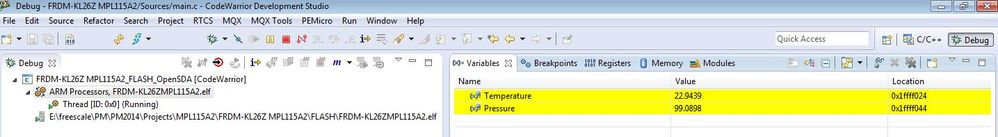## pressure sensor MPL115A2 with FRDM-KL26Z

cancel
Showing results for
Search instead for
Did you mean:
SOLVED

## pressure sensor MPL115A2 with FRDM-KL26Z

Jump to solution
392 ViewsContributor II

HI, I am working on a demo on smart inhaler. Does anyone have the source code for pressure sensor MPL115A2? I am trying to use it with FRDM-KL26Z. Any help on where can I find it would be appreciated.

Labels (1)
• ### Pressure Sensors

Tags (3)
1 Solution
121 ViewsNXP TechSupport

Hi Parth,

Attached you can find the source code for the FRDM-KL26Z board and the MPL115A2 pressure sensor on the KITMPL115A2I2C board. It is written in the CW for MCU's v10.6 and uses the I2C1 module (PTC1 and PTC2 pins) for I2C communication. Both the pressure and temperature are calculated in the infinite while loop:

```while(1)
{
I2C_WriteRegister(MPL115A2_I2C_ADDRESS, CONVERT_REG, 0x00);        // Start pressure and temperature conversion

Pause(0x17B2);        // Wait ~4ms (3ms is a minimum delay)

I2C_ReadMultiRegisters(MPL115A2_I2C_ADDRESS, Padc_MSB_REG, 4, RawPressureTempData);        // Read raw pressure and temperature data

// Temperature calculation
Tadc = ((uint16_t) (RawPressureTempData<<8 | RawPressureTempData)) >> 6;
Temperature = (605.75 - Tadc) * 0.186916;

// Pressure calculation
Padc = ((uint16_t) (RawPressureTempData<<8 | RawPressureTempData)) >> 6;
c12x2 = (((float) C12) / 4194304) * Tadc;
a1 = (((float) B1) / 8192) + c12x2;
a1x1 = a1 * Padc;
y1 = (((float) A0) / 8) + a1x1;
a2x2 = (((float) B2) / 16384) * Tadc;
Pcomp = y1 + a2x2;
Pressure = (Pcomp * 65 / 1023) + 50;

Pause(0xFFFF);
}

```

and can be watched in the "Variables" window on the top right of the Debug perspective:I hope it helps.

Regards,

Tomas

PS: If my answer helps to solve your question, please mark it as "Correct" or “Helpful”. Thank you.

2 Replies
122 ViewsNXP TechSupport

Hi Parth,

Attached you can find the source code for the FRDM-KL26Z board and the MPL115A2 pressure sensor on the KITMPL115A2I2C board. It is written in the CW for MCU's v10.6 and uses the I2C1 module (PTC1 and PTC2 pins) for I2C communication. Both the pressure and temperature are calculated in the infinite while loop:

```while(1)
{
I2C_WriteRegister(MPL115A2_I2C_ADDRESS, CONVERT_REG, 0x00);        // Start pressure and temperature conversion

Pause(0x17B2);        // Wait ~4ms (3ms is a minimum delay)

I2C_ReadMultiRegisters(MPL115A2_I2C_ADDRESS, Padc_MSB_REG, 4, RawPressureTempData);        // Read raw pressure and temperature data

// Temperature calculation
Tadc = ((uint16_t) (RawPressureTempData<<8 | RawPressureTempData)) >> 6;
Temperature = (605.75 - Tadc) * 0.186916;

// Pressure calculation
Padc = ((uint16_t) (RawPressureTempData<<8 | RawPressureTempData)) >> 6;
c12x2 = (((float) C12) / 4194304) * Tadc;
a1 = (((float) B1) / 8192) + c12x2;
a1x1 = a1 * Padc;
y1 = (((float) A0) / 8) + a1x1;
a2x2 = (((float) B2) / 16384) * Tadc;
Pcomp = y1 + a2x2;
Pressure = (Pcomp * 65 / 1023) + 50;

Pause(0xFFFF);
}

```

and can be watched in the "Variables" window on the top right of the Debug perspective:I hope it helps.

Regards,

Tomas

PS: If my answer helps to solve your question, please mark it as "Correct" or “Helpful”. Thank you.

121 ViewsContributor II

Thanks a lot.

It is working.